# Variational Autoencoder with Tensorflow – XIV – Change of variational model parameters at inference time

In my last post of this series I compared a Variational Autoencoder [VAE] with only a tiny amount of KL-loss to a standard Autoencoder [AE]. See the links at

Variational Autoencoder with Tensorflow – XIII – Does a VAE with tiny KL-loss behave like an AE? And if so, why?

Both the Keras models for the VAE and the AE were trained on the CelebA dataset of images of human heads. We found a tight similarity regarding the clustering of predicted data points for the training and test data in the latent space. In addition the VAE with tiny KL loss failed to reconstruct reasonable human face images from arbitrarily chosen points in the latent space. Just as a standard AE does. In forthcoming posts we will continue to study the relation between VAEs and AEs.

But in this post I want to briefly point out an interesting technical problem which may arise when you start to tests predictions for certain data samples after a training phase. Your Encoder or Decoder models may include parameters which you want to experiment with when predicting results for interesting input data. This raises the question whether we can vary such parameters at inference time. Actually, this was not quite as easy as it seemed to be when I started with respective experiments. To perform them I had to learn two aspects of Keras models I had not been aware of before.

# How to switch of the z-point variation at inference time?

In my particular case the starting point was the following consideration:

At inference time there is no real need for using the logvar-based variation around mu-values predicted by the Encoder.

The variation in z-point values in VAEs is done by adding a statistical value to mu-values. The added term is based on a log_var value multiplied by a statistically fluctuating factor “epsilon”, which comes from a normal Gaussian distribution around zero. mu, therefore, is the center of a distribution a specific input tensor is mapped to in latent space for consecutive predictions. The mu- and log_var-values depend on weights of two dense layers of the Encoder and thus indirectly on the optimization during training.

But while the variation is essential during training one may NOT regard it necessary for predictions. During inference we may in some experiments have good reasons to only refer to the central mu value when predicting a z-point in latent space. For test and analysis purposes it could be interesting to omit the log_var contribution.

The question then is: How we can switch off the log_var component for the Encoder’s predictions, i.e. predictions of our Keras based encoder model?

One idea is to include variables of a Python class hosting the Keras models for the Encoder, Decoder and the composed VAE in the function for the calculation of z-point vectors.

# The mu-, logvar and sampling layers of the VAE’s Encoder model encapsulated in a Python class

During this post series we have encapsulated the code for Encoder, Decoder and resulting VAE models in a Python class. Remember that the Encoder produced its output, namely z-points in the latent space via two “dense” Keras layers and a sampling layer (based on a Keras Lambda-layer). The dense layers followed a series of convolutional layers and gave us mu and log_var values. The Lambda-layer produced the eventual z-point-vector including the variation. In my case the code fragments for the layers look similar to the following ones:

```# .... Layer model of the Encoder part
...
...     # Definition of an input layer and multiple Conv2D layers
...
# "Variational" part - 2 Dense layers for a statistical distribution of z-points
self.mu      = Dense(self.z_dim, name='mu')(x)
self.log_var = Dense(self.z_dim, name='log_var')(x)
# Layer to provide a z_point in the Latent Space for each sample of the batch
self._encoder_output = Lambda(z_point_sampling, name='encoder_output')([self.mu, self.log_var])
...
# The Encoder model
self.encoder = Model(inputs=self._encoder_input, outputs=[self._encoder_output, self.mu, self.log_var], name="encoder")
...
```

The “self” refers to a class “MyVariationalAutoencoder” comprising the Encoder’s, Decoder’s and the VAE’s model and layer structures. See for details and explained code fragments of the class e.g. the posts around Variational Autoencoder with Tensorflow 2.8 – X – VAE application to CelebA images.

The sampling is in my case done by a function “z_point_sampling”:

```        def z_point_sampling(args):
'''
A point in the latent space is calculated statistically
around an optimized mu for each sample
'''
mu, log_var = args # Note: These are 1D tensors !
epsilon = B.random_normal(shape=B.shape(mu), mean=0., stddev=1.)
return mu + B.exp(log_var / 2.) * epsilon * self.enc_v_eps_factor
```

You see that this function uses a class member variable “self.enc_v_eps_factor”.

# Switch the variation with log_var on and off for predictions?

Our objective is to switch the log_var contribution on or off for input certain images or batches of such images fed into the Encoder. For this purpose we could in principle use the variable “self.enc_v_eps_factor” as a kind of boolean switch with values of either 0.0 or 1.0. To set the variable I had defined two class methods:

```    def set_enc_to_predict(self):
self.enc_v_eps_factor = 0.0

def set_enc_to_train(self):
self.enc_v_eps_factor = 1.0
```

The basic idea was that the sampling function would pick the value of enc_v_eps_factor given at the runtime of a prediction, i.e. at inference time. This assumption was, however, wrong. At least in a certain sense.

# Is a class variable change impacting a layer output noted during consecutive predictions of a Keras model?

Let us assume that we have instantiated our class and assigned it to a Python variable MyVae. Let us further assume the the comprised Keras models are referenced by variables

• MyVae.encoder (for the Encoder part),
• MyVae.decoder (for the Decoder part)
• and MyVae.model (for the full VAE-model).

We do not care about further details of the VAE (consisting of the Encoder, the Decoder and GradientTape based cost control). But we should not forget that all the models’ layers and their weights determine cost functions derivatives and are therefore targets of the optimization performed during training. All factors determining gradients and value calculation with given weights are encoded with the compilation of a Keras model – for training purposes [using model.fit() with a Keras model], but as well for predictions! Without a compiled Keras model we cannot use model.predict().

This means: As soon as you have a compiled Keras model almost and load the weight-values saved after a a sufficient number of training epochs everything is settled for inference and predictions. Including the present value of self.enc_v_eps_factor. At compile time?

Well, thinking about it a bit more in detail from a developer perspective tells us:
The compilation would in principle not prevent the use of a changed variable at the run-time of a prediction. But on the other hand side we also have the feeling that Keras must do something to make training (which also requires predictions in the sense of a forward pass) and later raw predictions for batches at inference time pretty fast. Intermediate functionality changes would hamper performance – if only for the reason that you have to watch out for such changes.

So, it is natural to assume that Keras would keep any factors in the Lambda-layer taken from a class variable constant after compilation and during training or inference, i.e. predictions.

If this assumption were true then chain of actions AFTER a training of a VAE model (!) like

Define a Keras based VAE-model with a sampling layer and a factor enc_v_eps_factor = 1   =>   compile it (including the sub-models for the Encoder and Decoder)   =>   load weight parameters which had been saved after training   =>   switch the value of the class variable enc_v_eps_factor to 0.0   =>   Load an image or image batch for prediction

would probably NOT work as expected. To be honest: This is “wisdom” derived after experiments which did not give me the naively expected results.

Indeed, some first simple experiments showed: The value of enc_v_eps_factor (e.g. enc_v_eps_factor = 1) which was given at compile-time was used during all following prediction calculations, e.g. for a particular image in tensorial form. So, a command sequence like

```MyVae.set_enc_to_train()
MyVae.encoder.compile()
...
# Load weights from a set path
...

z_point, mu, log_var = MyVae.encoder.predict(img1)
print(z_point, mu)
MyVae.set_enc_to_predict(self)
z_point, mu, log_var = MyVae.encoder.predict(img1)
```

did not give different results. Note, that I did not change the value of enc_v_eps_factor between compile time and the first call for a prediction.

Let us look at the example in more detail.

# A concrete example

After a full training of my VAE on CelebA for 24 epochs I checked for a maximum log_var-value. Non-negligible values may indeed occur for certain images and special z-vector components for some images despite the tiny KL-loss. And indeed such a value occurred for a very special (singular) image with two diagonal black border stripes on the left and right of the photographed person’s head. I do not show the image due to digital rights concerns. But let us look at the predicted z, mu and log_var values (for a specific z-point vector component) of this image. Before I compiled the VAE model I had set enc_v_eps_factor = 1.0 :

```# Set the learning rate and COMPILE the model
# ~~~~~~~~~~~~~~~~~~~~~~~~~~~~~~~~~~~~~~~~~~~~
learning_rate = 0.0005

# The following is only required for compatibility reasons
b_old_optimizer = True

# Set enc_v_eps_factor to 1.0
MyVae.set_enc_to_train()

# Separate Encoder compilation
# - does not harm the compilation of the full VAE-model, but is useful to avoid later trace warnings after changes
MyVae.encoder.compile()

# Compilation of the full VAE model (Encoder, Decoder, cost functions used by GradientTape)
MyVae.compile_myVAE(learning_rate=learning_rate, b_old_optimizer = b_old_optimizer )
...

# Load weights from a set path
...
# start predictions
...
```

with

```    def compile_myVAE(self, learning_rate, b_old_optimizer = False):
# Version 1.1 of 221212
# Forced to special handling of the optimizer for data resulting from training before TF 11.2 , due to warnings:
#      ValueError: You are trying to restore a checkpoint from a legacy Keras optimizer into a v2.11+ Optimizer,
#      which can cause errors. Please update the optimizer referenced in your code to be an instance

# Optimizer handling
# ~~~~~~~~~
if b_old_optimizer:
else:
....

# Solution type with train_step() and GradientTape()
if self.solution_type == 3:
self.model.compile(optimizer=optimizer)

```

Details of the compilation are not important – though you may be interested in the fact that training data saved after a training based on Python module version < 11.2 of TF 2 requires a legacy version of the optimizer when later using a module version ≥ 11.2 (corresponding to TF V2.11 and above).

However, the really important point is that the compilation is done given a certain value of enc_v_eps_factor = 1.

Then we load the image in form a prepared training batch with just one element set and provide it to the predict() function of the Keras model for the Encoder. We perform two predictions and ahead of the second one we change the value of enc_v_eps_factor to 0.0:

```# Choose an image
j = 123223
img = x_train[j] # this has already a tensor compatible format
img_list = []
img_list.append(img)
tf_img = tf.convert_to_tensor(img_list)

# Encoder prediction
z_points, mu, logvar  = MyVae.encoder.predict(tf_img)

print(z_points)
print(mu)
print(logvar)

# !!!! Set enc_v_eps_factor to 0.0 !!!!
MyVae.set_enc_to_predict()

# New Encoder prediction
z_points, mu, logvar  = MyVae.encoder.predict(tf_img)

print()
print()

print(z_points)
print(mu)
print(logvar)

```

The result is:

```...
2.637196
-0.141142
3.3761873
...
-0.2085279
-0.141142
3.3761873
```

The result depends on statistical variations for the factor epsilon (Gaussian statistics; see the sampling function above).

But the central point is not the deviation for the two different prediction calls. The real point is that we have used MyVae.set_enc_to_predict() ahead of the second prediction and, yet, the values for mu and log_var for the special z_point-component (230; out of 256 components) were NOT identical. I.e. the variable value enc_v_eps_factor = 1.0, which we set before the compilation, was used during both of our prediction calculations!

# Can we just recompile between different calls to model.predict() ?

The experiment described above seems to indicate that the value for the class variable enc_v_eps_factor given at compile time is used during all consecutive predictions. We could, of course, enforce a zero variation for all predictions by using MyVae.set_enc_to_predict() ahead of the compilation of the Encoder model. But this would not give us no flexibility to switch the log_var contribution off ahead of predictions for some special images and then turn it on again for other images.

But the is simple – if we need not do this switching permanently: We just recompile the Encoder model!

Compilation does not take much time for Encoder models with only a few (convolutional and dense) layers. Let us test this by modifying the code above:

```# Choose an image
j = 123223
img = x_train[j] # this has already a tensor compatible format
img_list = []
img_list.append(img)
tf_img = tf.convert_to_tensor(img_list)

# Set enc_v_eps_factor to 0.0
MyVae.set_enc_to_predict()
# !!!!
MyVae.encoder.compile()

# Encoder prediction
z_points, mu, logvar  = MyVae.encoder.predict(tf_img)
# Decoder prediction - just for fun
reco_list = MyVae.decoder.predict(z_points) # just for fun
print(z_points)
print(mu)
print(logvar)

print()
print()

# Set enc_v_eps_factor back to 1.0
MyVae.set_enc_to_train()
MyVae.encoder.compile()

# New Encoder prediction
z_points, mu, logvar  = MyVae.encoder.predict(tf_img)

print(z_points)
print(mu)
print(logvar)
```

We get

```Shape of img_list =  (1, 96, 96, 3)
eps_fact =  0.0
1/1 [==============================] - 0s 280ms/step
1/1 [==============================] - 0s 18ms/step
Shape of reco_list =  (1, 96, 96, 3)

-0.141142
-0.141142
3.3761873

eps_fact =  1.0
1/1 [==============================] - 0s 288ms/step

-0.63676023
-0.141142
3.3761873
```

This is exactly what we want!

# The function for the prediction step of a Keras model is cached at inference time …

The example above gave us the impression that it could be the compilation of a model which “settles” all of the functionality used during predictions, i.e. at inference time. Actually, this is no quite true.

The documentation on a Keras model helped me to get a better understanding. Near the section on the method “predict()” we find some other interesting functions. A look at the remarks on “predict_step()“, reveals (quotation)

```The logic for one inference step.

This method can be overridden to support custom inference logic. This method is called by Model.make_predict_function.

This method should contain the mathematical logic for one step of inference. This typically includes the forward pass.
```

This leads us to the function “make_predict_function()” for Keras models. And there we find the following interesting remarks – I quote:

```This method can be overridden to support custom inference logic. This method is called by Model.predict and Model.predict_on_batch.

Typically, this method directly controls tf.function and tf.distribute.Strategy settings, and delegates the actual evaluation logic to Model.predict_step.

This function is cached the first time Model.predict or Model.predict_on_batch is called. The cache is cleared whenever Model.compile is called. You can skip the cache and generate again the function with force=True.
```

Ah! The function predict_step() normally covers the forward pass through the network and “make_predict_function()” caches the resulting (function) object at the first invocation of model.predict(). And the respective cache is not cleared automatically.

So, what really may have hindered my changes of the sampling functionality at inference time is a cache filled at the first call to encoder.predict()!

Let us test this!

# Changing the sampling parameters after compilation, but before the first call of encoder.predict()

If our suspicion is right we should be able to set up the model from scratch again, compile it, use MyVae.set_enc_to_predict() and afterward call MyVae.encoder.predict() – and get the same values for mu and z_point.

So we do something like

```# Build encoder according to layer parameters
MyVae._build_enc()
# Build decoder according to layer parameters
MyVae._build_dec()
# Build the VAE-model
MyVae._build_VAE()
...

# Set variable to 1.0
MyVae.set_enc_to_train()

# Compile
learning_rate = 0.0005
b_old_optimizer = True
MyVae.compile_myVAE(learning_rate=learning_rate, b_old_optimizer = b_old_optimizer )
MyVae.encoder.compile()   # used to prevent retracing delays - when later changing encoder variables

...
# Load weights from a set path
...

# preparation if the selected img.
...
...

MyVae.set_enc_to_predict()
print("eps_fact = ", MyVae.enc_v_eps_factor)
# Note: NO recompilation is done !

# First prediction
z_points, mu, logvar  = MyVae.encoder.predict(tf_img)
print()
print(z_points)
print(mu)
print(logvar)
..
```

Note that the change of enc_v_eps_factor ahead of the first call of predict(). And, indeed:

```Shape of img_list =  (1, 96, 96, 3)
eps_fact =  0.0
...
-0.141142
-0.141142
3.3761873
```

# Use make_predict_function(force=True) to clear and refill the cache for predict_step() and its forward pass functionality

The other option the documentation indicates is to use the function make_predict_function(force=True).
This leads to yet another experiment:

```# img preparation
....

# Set enc_v_eps_factor to 1.0
MyVae.set_enc_to_train()
MyVae.encoder.compile()
print("eps_fact = ", MyVae.enc_v_eps_factor)

# Encoder prediction
z_points, mu, logvar  = MyVae.encoder.predict(tf_img)
print(z_points)
print(mu)
print(logvar)

print()
print()

# Set enc_v_eps_factor to 0.0
MyVae.set_enc_to_predict()
# !!!!
MyVae.encoder.make_predict_function(
force=True
)
print("eps_fact = ", MyVae.enc_v_eps_factor)

# Encoder prediction
z_points, mu, logvar  = MyVae.encoder.predict(tf_img)
print(z_points)
print(mu)
print(logvar)
```

We get

```...
eps_fact =  1.0
1/1 [==============================] - 0s 287ms/step

-5.5451365
-0.141142
3.3761873

eps_fact =  0.0
1/1 [==============================] - 0s 271ms/step

-0.141142
-0.141142
3.3761873

```

Yes, exactly as expected. This again shows us that it is the cache which counts after the first call of model.predict() – and not the compilation of the Keras model (for the Encoder) !

# Other approaches?

The general question of changing parameters at inference time also triggers the question whether we may be able to deliver parameters to the function model.predict() and transfer them further to customized variants of predict_step(). I found a similar question at stack overflow
Passing parameters to model.predict in tf.keras.Model

However, the example there was rather special – and I did not apply the lines of thought explained there to my own case. But the information given in the answer may still be useful for other readers.

# Conclusion

In this post we have seen that we can change parameters influencing the forward pass of a Keras model at inference time. We saw, however, that we have to clear and fill a cache to make the changes effective. This can be achieved by

• either applying a recompilation of the model
• or enforcing a clearance and refilling of the cache for the model’s function predict_step().

In the special case of a VAE this allows for deactivating and re-activating the logvar-dependent statistical variation of the z-points a specific image is mapped to by the Encoder model during predictions. This gives us the option to focus on the central mu-dependent position of certain images in the latent space during experiments at inference time.

In the next post of this series we shall have a closer look at the filamental structure of the latent space of a VAE with tiny KL loss in comparison to the z-space structure of a VAE with sufficiently high KL loss.

Ceterum censeo: The worst fascist, war criminal and killer living today is the Putler. He must be isolated at all levels, be denazified and sooner than later be imprisoned. Somebody who orders the systematic destruction of civilian infrastructure must be fought and defeated because he is a permanent danger to basic principles of humanity – not only in Europe. Long live a free and democratic Ukraine!

## Variational Autoencoder with Tensorflow – XIII – Does a VAE with tiny KL-loss behave like an AE? And if so, why?

### Image

This post continues my series on Variational Autoencoders [VAE] with some considerations regarding a VAE whose settings allow for only a tiny amount of the so called Kullback-Leibler [KL] loss.

So far, most of the posts in this series have covered a variety of methods (provided by Tensorflow and Keras) to control the KL loss. One of the previous posts (XI) provided (indirect) evidence that also GradientTape()-based methods for KL-loss calculation work as expected. In stark contrast to a standard Autoencoder [AE] our VAE trained on CelebA data proved its ability to reconstruct humanly interpretable images from random z-points (or z-vectors) in the latent space. Provided that the z-points lie within a reasonable distance to the origin.

We could leave it at that. One of the basic motivations to work with VAEs is to use the latent space “creatively”. This requires that the data points coming from similar training images should fill the latent space densely and without gaps between clusters or filaments. We have obviously achieved this objective. Now we could start to do funny things like to combine reconstruction with vector arithmetic in the latent space.

But to look a bit deeper into the latent space may give us some new insights. The central point of the KL-loss is that it induces a statistical element into the training of AEs. As a consequence a VAE fills the so called “latent space” in a different way than a simple AE. The z-point distribution gets confined and areas around z-points for meaningful training images are forced to get broader and overlap. So two questions want an answer:

• Can we get more direct evidence of what the KL-loss does to the data distribution in latent space?
• Can we get some direct evidence supporting the assumption that most of the latent space of an AE is empty or only sparsely populated? in contrast to a VAE’s latent space?

Therefore, I thought it would be funny to compare the data organization in latent space caused by an AE with that of a VAE. But to get there we need some solid starting point. If you consider a bit where you yourself would start with an AE vs. VAE comparison you will probably come across the following additional and also interesting questions:

• Can one safely assume that a VAE with only a very tiny amount of KL-loss reproduces the same z-point distribution vs. radius which an AE would give us?
• In general: Can we really expect a VAE with a very tiny Kullback-Leibler loss to behave as a corresponding AE with the same structure of convolutional layers?

The answers to all these questions are the topics of this post and a forthcoming one. To get some answers I will compare a VAE with a very small KL-loss contribution with a similar AE. Both network types will consist of equivalent convolutional layers and will be trained on the CelebA dataset. We shall look at the resulting data point density distribution vs. radius, clustering properties and the ability to create images from statistical z-points.

This will give us a solid base to proceed to larger and more natural values of the KL-loss in further posts. I got some new insights along this path and hope the presented data will be interesting for the reader, too.

Below and in following posts I will sometimes call the target space of the Encoder also the “z-space“.

# CelebA data to fill the latent vector-space

The training of an AE or a VAE occurs in a self-supervised manner. A VAe or an AE learns to create a point, a z-point, in the latent space for each of the training objects (e.g. CelebA images). In such a way that the Decoder can reconstruct an object (image) very close to the original from the z-point’s coordinate data. We will use the “CelebA” dataset to study the KL-impact on the z-point distribution.CelebA is more challenging for a VAE than MNIST. And the latent space requires a substantially higher number of dimensions than in the MNIST case for reasonable reconstructions. This makes things even more interesting.

The latent z-space filled by a trained AE or VAE is a multi-dimensional vector space. Meaning: Each z-point can be described by a vector defining a position in z-space. A vector in turn is defined by concrete values for as many vector components as the z-space has dimensions.

Of course, we would like to see some direct data visualizing the impact of the KL-loss on the z-point distribution which the Encoder creates for our training data. As we deal with a multidimensional vector space we cannot plot the data distribution. We have to simplify and somehow get rid of the many dimensions. A simple solution is to look at the data point distribution in latent space with respect to the distance of these points from the origin. Thereby we transform the problem into a one-dimensional one.

More precisely: I want to analyze the change in numbers of z-points within “radius“-intervals. Of course, a “radius” has to be defined in a multidimensional vector space as the z-space. But this can easily be achieved via an Euclidean L2-norm. As we expect the KL loss to have a confining effect on the z-point distribution it should reduce the average radius of the z-points. We shal later see that this is indeed the case.

Another simple method to reduce dimensions is to look at just one coordinate axis and the data distribution for the calculated values in this direction of the vector space. Therefore, I will also check the variation in the number of data points along each coordinate axis in one of the next posts.

A look at clustering via projections to a plane may be helpful, too.

# The expected similarity of a VAE with tiny KL-loss to an AE is not really obvious

Regarding the answers to the 3rd and 4th questions posed above your intuition tells you: Yes, you probably can bet on a similarity between a VAE with tiny KL-loss and an AE.

But when you look closer at the network architectures you may get a bit nervous. Why should a VAE network that has many more degrees of freedom than an AE not use both of its layers for “mu” and “logvar” to find a different distribution solution? A solution related to another minimum of the loss hyperplane in the weight configuration space? Especially as this weight-related space is significantly bigger than that of a corresponding AE with the same convolutional layers?

The whole point has to do with the following facts: In an AE’s Encoder the last flattening layer after the Conv2D-layers is connected to just one output layer. In a VAE, instead, the flattening layer feeds data into two consecutive layers (for mu and logvar) across twice as many connections (with twice as many weight parameters to optimize).

In the last post of this series we dealt with this point from the perspective of VRAM consumption. Now, its the question in how far a VAE will be similar to an AE for a tiny KL-loss.

Why should the z-points found be determined only by mu-values and not also by logvar-values? And why should the mu values reproduce the same distribution as an AE? At least the architecture does not guarantee this by any obvious means …

Well, let us look at some data.

# Structure of an AE for CelebA and its total loss after some epochs

Our test AE contains the same simple sequence of four Conv2D layers per Encoder and four 4 Conv2DTranspose layers as our VAE. See the AE’s Encoder layer structure below.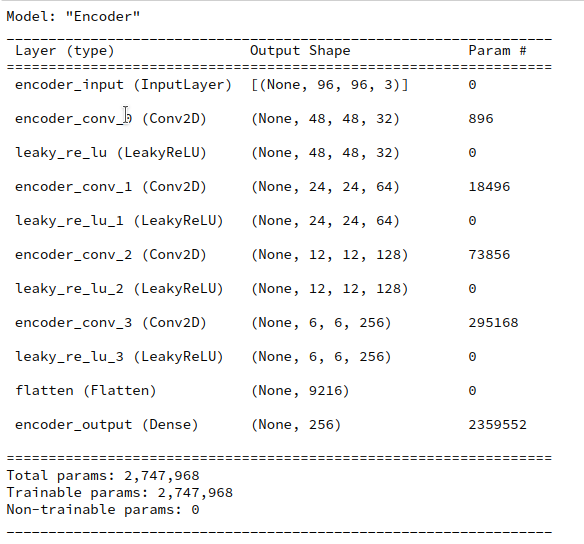A difference, however, will be that I will not use any BatchNormalizer layers in the AE. But as a correctly implemented BatchNormalization should not affect the representational powers of a VAE network for very principle reasons this should not influence the comparison of the final z-point distribution in a significant way.

I performed an AE training run for 170,000 CelebA training images over 24 epochs. The latent space has a dimension if z_dim=256. (This relatively low number of dimensions will make it easier for a VAE to confine z_points around the origin; see the discussion in previous posts).

The resulting total loss of our AE became ca. 0.49 per pixel. This translates into a total value of

AE total loss on Celeb A after 24 epochs (for a step size of 0.0005): 4515

This value results from a summation over all geometric pixels of our CelebA images which were downsized to 96×96 px (see post IX). The given value can be compared to results measured by our GradientTape()-based VAE-model which delivers integrated values and not averages per pixel.

This value is significantly smaller than values we would get for the total loss of a VAE with a reasonably big KL-loss of contribution in the order of some percent of the reconstruction loss. A VAE produces values around 4800 up to 5000. Apparently, an AE’s Decoder reconstructs originals much better than a VAE with a significant KL-loss contribution to the total loss.

But what about a VAE with a very small KL-loss? You will get the answer in a minute.

# Where does a standard Autoencoder [AE] place the z-points for CelebA data?

We can not directly plot a data point distribution in a 256-dimensional vector-space. But we can look at the data point density variation with a calculated distance from the origin of the latent space.

The distance R from the origin to the z-point for each image can be measured in terms of a L2 (= Euclidean) norm of the latent vector space. Afterward it is easy to determine the number of images within all radius intervals with e.g. a length of 0.5 e.g. between radii R

0  <  R  <  35 .

We perform the following steps to get respective numbers. We let the Encoder of our trained AE predict the z-points of all 170,000 training data

```z_points  = AE.encoder.predict(data_flow)
```

data_flow was created by a Keras DataImageGenerator to send batches of training data to the GPU (see the previous posts).

Radius values are then calculated as

```print("NUM_Images_Train = ", NUM_IMAGES_TRAIN)
for i in range(0, NUM_IMAGES_TRAIN):
sq = np.square(z_points[i])
sqrt_sum_sq = math.sqrt(sq.sum())
```

The numbers vs. radius relation then results from:

```li_rad      = []
int_width = 0.5
for i in range(0,70):
low   = int_width * i
high  = int_width * (i+1)
num   = np.count_nonzero( (ay_rad_z >= low) & (ay_rad_z < high ) )
```

The resulting curve is shown below: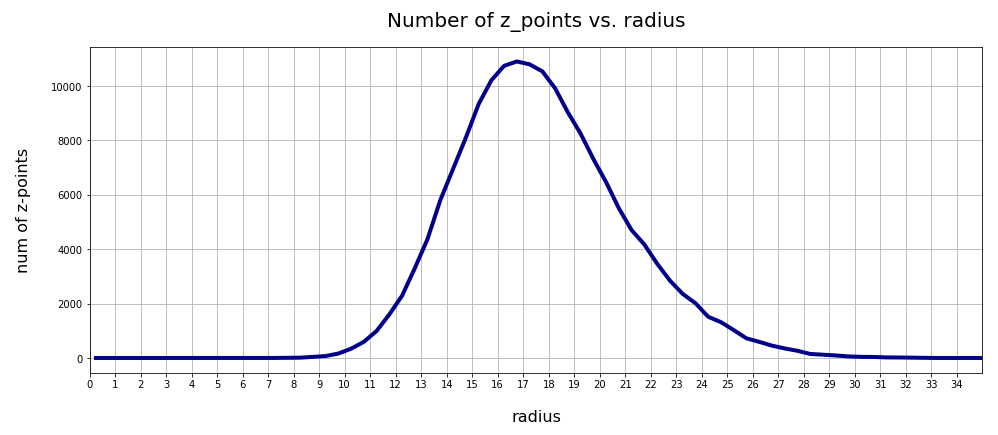There seems to be a peak around R = 16.75. So, yet another question arises:

>What is so special about the radius values of 16 or 17 ?

We shall return to this point in the next post. For now we take this result as god-given.

# Clustering of CelebA z-point data in the AE’s latent space?

Another interesting question is: Do we get some clustering in the latent space? Will there be a difference between an AE and a VAE?

A standard method to find an indication of clustering is to look for an elbow in the so called “inertia” curve for different assumed numbers of clusters. Below you find an inertia plot retrieved from the z-point data with the help of MiniBatchKMeans.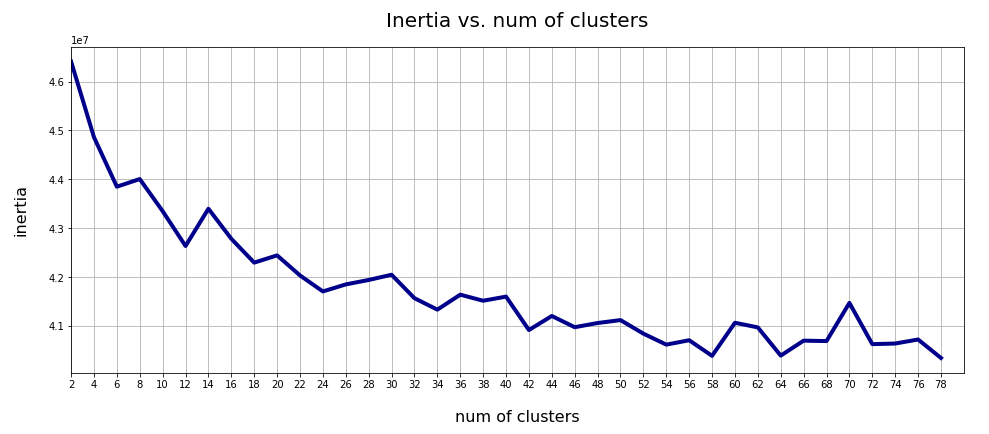This result was achieved for data taken at every second value of the number of clusters “num_clus” between 2 ≤ num_clus ≤ 80. Unfortunately, the result does not show a pronounced elbow. Instead the variation at some special cluster numbers is relatively high. But, if we absolutely wanted to define a value then something between 38 and 42 appears to be reasonable. Up to that point the decline in inertia is relatively smooth. But do not let you get misguided – the data depend on statistics and initial cluster values. When you change to a different calculation you may get something like the following plot with more pronounced spikes: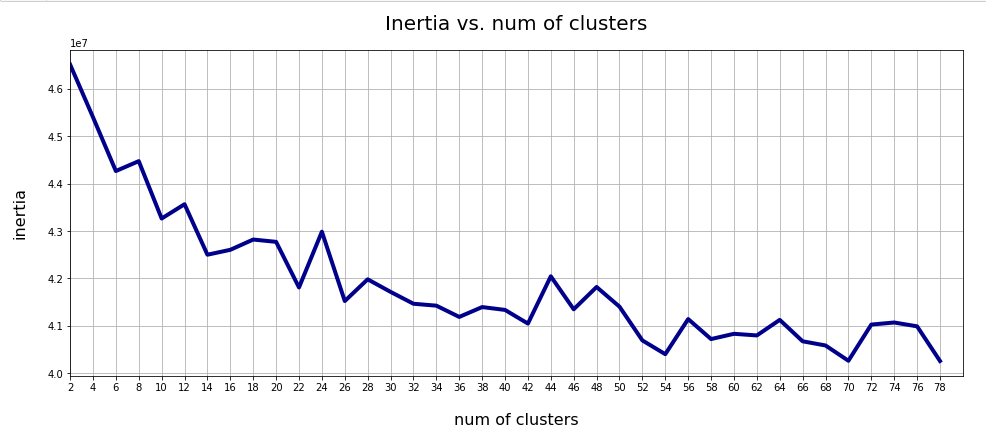This is always as sign that the clustering is not very clear – and that the clusters do not have a significant distance, at least not in all coordinate directions. Filamental structures will not be honored well by KMeans.

Nevertheless: A value of 40 is reasonable as we have 40 labels coming with the CelebA data. I.e. 40 basic features in the face images are considered to be significant and were labeled by the creators of the dataset.

## t-SNE projections

We can also have a look at a 2-dimensional t-SNE-projection of the z-point distribution. The plots below have been produced with different settings for early exaggeration and perplexity parameters. The first plot resulted from standard parameter values for sklearn’s t-SNE variant.

```tsne = TSNE(n_components=2, early_exaggeration=12, perplexity=30, n_iter=1000)
```

Other plots were produced by the following setting:

```tsne = TSNE(n_components=2, early_exaggeration=16, perplexity=10, n_iter=1000)
```

Below you find some plots of a t-SNE-analysis for different numbers and different adjusted parameters for the resulting scatter plot. The number of statistically chosen z-point varies between 20,000 and 140,000.

Number of statistical z-points: 20,000 (non-standard t-SNE-parameters)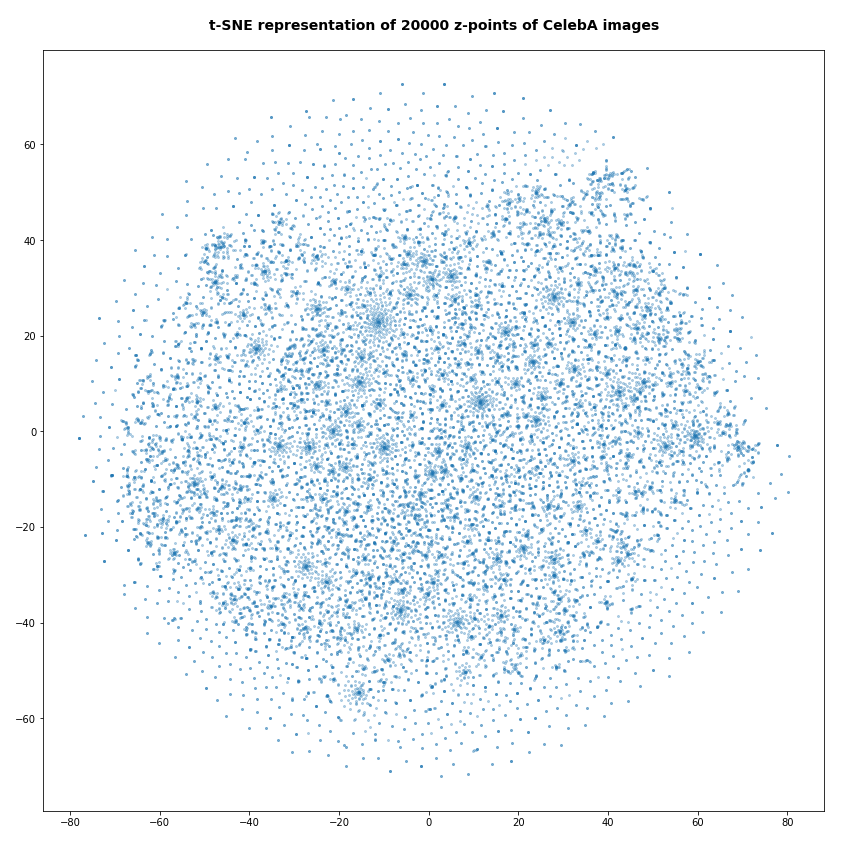Actually we see some indication of clustering, though it is not very pronounced. The clusters in the projection are not separated by clear and broad gaps. Of course a 2-dimensional projection can not completely visualize the original separations in a 256-dim space. However, we get the impression that clusters are located rather close to each other. Remember: We already know that almost all points are locates in a multidimensional sphere shell between 12 < R < 24. And more than 50% between 14 ≤ R ≤ 19.

However, how the actual distribution of meaningful z-points (in the sense of a recognizable face reconstruction) really looks like cannot be deduced from the above t-SNE analysis. The concentration of the z-points may still be one which follows thin and maybe curved filaments in some directions of the multidimensional latent space on relatively small or various scales. We shall get a much clearer picture of the fragmentation of the z-point distribution in an AE’s latent space in the next post of this series.

Number of statistical z-points: 80,000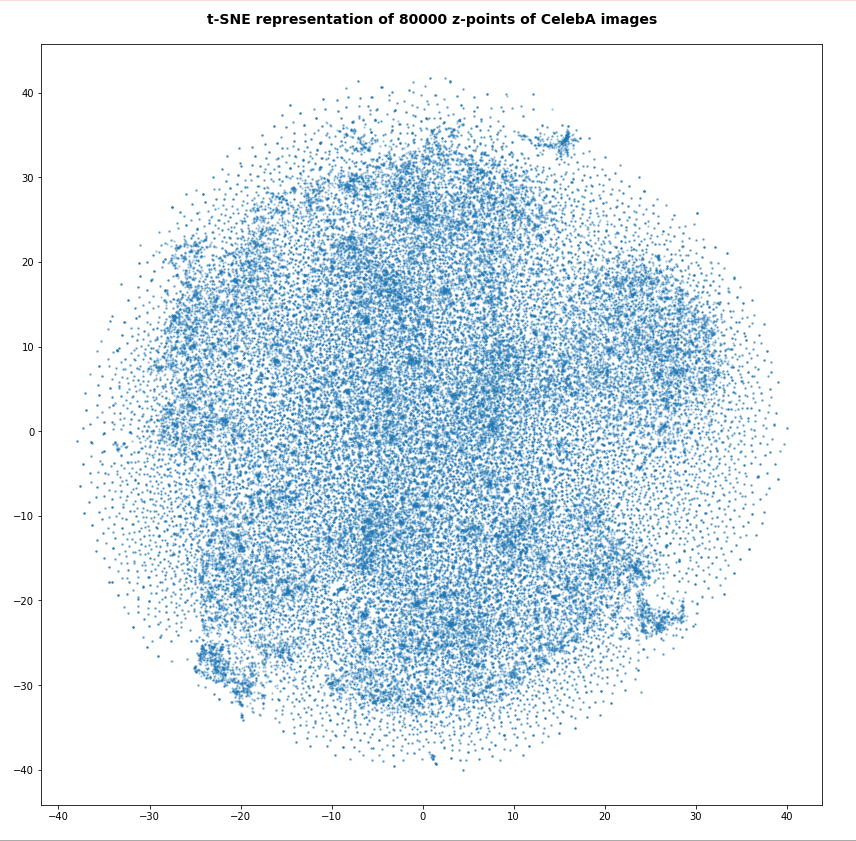For the higher number of selected z-points the room between some concentration centers appears to be filled in the projection. But remember: This may only be due to projection effects in the presently chosen coordinate system. Another calculation with the above non-standard data for perplexity and early_exaggeration gives us: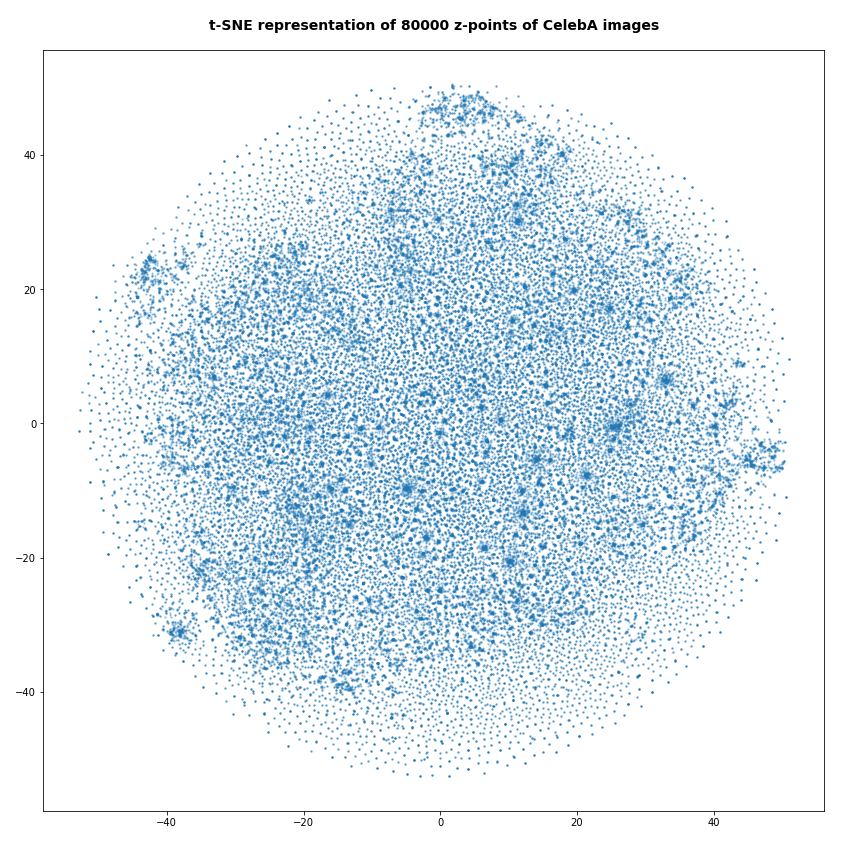Number of statistical z-points: 140,000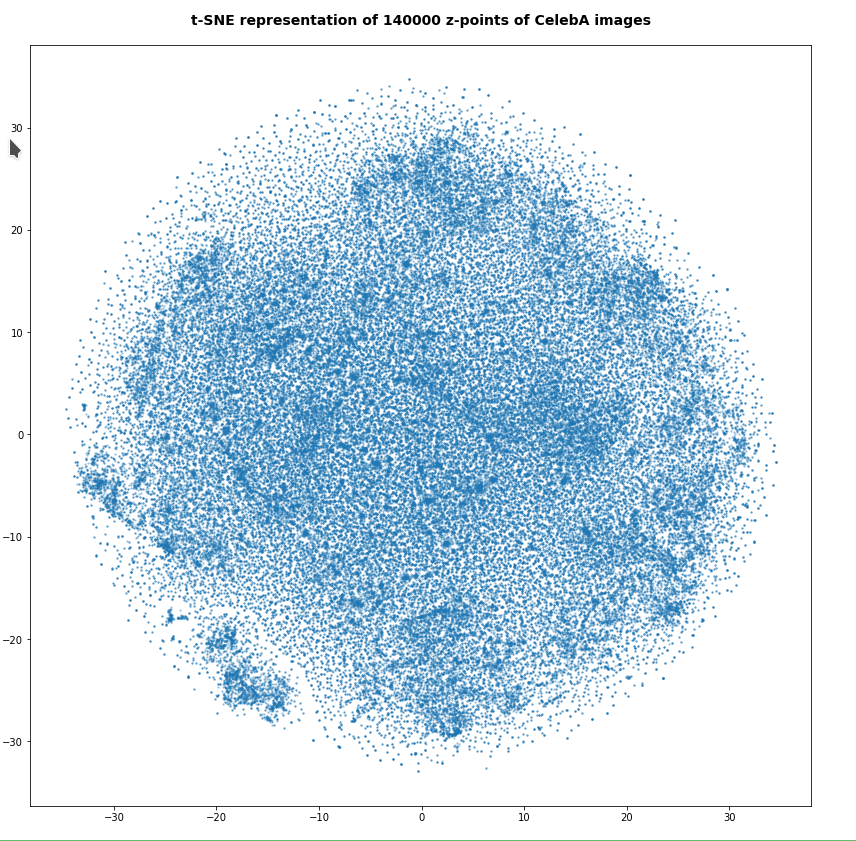Note that some islands appear. Obviously, there is at least some clustering going on. However, due to projection effects we cannot deduce much for the real structure of the point distribution between possible clusters. Even the clustering itself could appear due to overlapping two or more broader filaments along a projection line.

Whether correlations would get more pronounced and therefore could also be better handled by t-SNE in a rotated coordinate system based on a PCA-analysis remains to be seen. The next post will give an answer.

At least we have got a clear impression about the radial distribution of the z-points. And thereby gathered some data which we can compare to corresponding results of a VAE.

# Total loss of a VAE with a tiny KL-loss for CelebA data

Our test VAE is parameterized to create only a very small KL-loss contribution to the total loss. With the Python classes we have developed in the course of this post series we can control the ratio between the KL-loss and a standard reconstruction loss as e.g. BCE (binary-crossentropy) by a parameter “fact“.

For BCE

```fact = 1.0e-5
```

is a very small value. For a working VAE we would normally choose something like fact=5 (see post XI).

A value like 1.0e-5 ensures a KL loss around 0.0178 compared to a reconstruction loss of 4550, which gives us a ratio below 4.e-6. Now, what is a VAE going to do, when the KL-loss is so small?

For the total loss the last epochs produced the following values:

AE total loss on Celeb A after 24 epochs for a step size of 0.0005: 4,553

Output of the last 6 of 24 epochs.

```Epoch 1/6
1329/1329 [==============================] - 120s 90ms/step - total_loss: 4557.1694 - reco_loss: 4557.1523 - kl_loss: 0.0179
Epoch 2/6
1329/1329 [==============================] - 120s 90ms/step - total_loss: 4556.9111 - reco_loss: 4556.8940 - kl_loss: 0.0179
Epoch 3/6
1329/1329 [==============================] - 120s 90ms/step - total_loss: 4556.6626 - reco_loss: 4556.6450 - kl_loss: 0.0179
Epoch 4/6
1329/1329 [==============================] - 120s 90ms/step - total_loss: 4556.3862 - reco_loss: 4556.3682 - kl_loss: 0.0179
Epoch 5/6
1329/1329 [==============================] - 120s 90ms/step - total_loss: 4555.9595 - reco_loss: 4555.9395 - kl_loss: 0.0179
Epoch 6/6
1329/1329 [==============================] - 118s 89ms/step - total_loss: 4555.6641 - reco_loss: 4555.6426 - kl_loss: 0.0178

```

This is not too far away from the value of our AE. Other training runs confirmed this result. On four different runs the total loss value came to lie between

VAE total loss on Celeb A after 24 epochs: 4553 ≤ loss ≤ 4555 .

# VAE with tiny KL-loss – z-point density distribution vs. radius

Below you find the plot for the variation of the number density of z-points vs. radius for our VAE: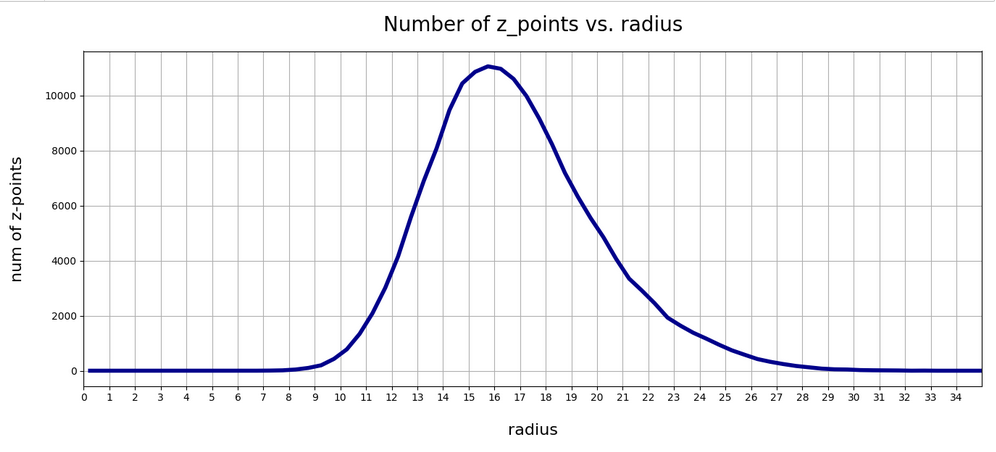Again, we get a maximum close to R = rad = 16. The maximum value lies a bit below the one found for a KL-loss-free AE. But all in all the form and width of the distribution of the VAE are very comparable to that of our test AE.

Can this result be reproduced?
Unfortunately not at a 100% of test runs performed. There are two main reasons:

1. Firstly, we can not be sure that a second minimum does not exist for a distribution of points at bigger radii. This may be the case both for the AE and the VAE!
2. Secondly, we have a major factor of statistical fluctuation in our game:
The epsilon value which scales the logvar-contribution to the loss in the sampling layer of the Encoder may in very seldom cases abruptly jump to an unreasonable high value. A Gaussian covers extreme values, although the chances to produce such a value are pretty small. and a Gaussian is invilved in the calculation of z-points by our VAE.

Remember that the z-point coordinates are calculated via the the mu and logvar tensors according to

```
z = mu + B.exp(log_var / 2.) * epsilon
```

See Variational Autoencoder with Tensorflow 2.8 – VIII – TF 2 GradientTape(), KL loss and metrics for respective code elements of the Encoder.

So, a lot depends on epsilon which is calculated as a statistically fluctuating quantity, namely as

```epsilon = B.random_normal(shape=B.shape(mu), mean=0., stddev=1.)
```

Is there a chance that the training process may sometimes drive the system to another corner of the weight-loss configuration space due to abrupt fluctuations? With the result for the z-point distribution vs. radius that it may significantly deviate from a distribution around R = 16? I think: Yes, this is possible!

From some other training runs I actually have an indication that there is a second minimum of the cost hyperplane with similar properties for higher average radius-values, namely for a distribution with an average radius at R ≈ 19.75. I got there after changing the initialization of the weights a bit.

Another indication that the cost surface has a relative rough structure and that extreme fluctuations of epsilon and a resulting gradient-fluctuation can drive the position of the network in the weight configuration space to some strange corners. The weight values there can result in different z-point distributions at higher average radii. This actually happened during yet another training run: At epoch 22 the Adam optimizer suddenly directed the whole system to weight values resulting in a maximum of the density distribution at R = 66 ! This appeared as totally crazy. At the same time the KL-loss also jumped to a much higher value.

When I afterward repeated the run from epoch 18 this did not happen again. Therefore, a statistical fluctuation must have been the reason for the described event. Such an erratic behavior can only be explained by sudden and extreme changes of z-point data enforcing a substantial change in size and direction of the loss gradient. And epsilon is a plausible candidate for this!

So far I had nothing in our Python classes which would limit the statistical variation of epsilon. The effects seen spoke for a code change such that we do not allow for extreme epsilon-values. I set limits in the respective part of the code for the sampling layer and its lambda function

```        # The following function will be used by an eventual Lambda layer of the Encoder
def z_point_sampling(args):
'''
A point in the latent space is calculated statistically
around an optimized mu for each sample
'''
mu, log_var = args # Note: These are 1D tensors !
epsilon = B.random_normal(shape=B.shape(mu), mean=0., stddev=1.)
if abs(epsilon) >= 5:
epsilon *= 5. / abs(epsilon)
return mu + B.exp(log_var / 2.) * epsilon * self.enc_v_eps_factor
```

This stabilized everything. But even without these limitations on average three out of 4 runs which I performed for the VAE ran into a cost minimum which was associated with a pronounced maximum of the z-point-distribution around R ≈ 16. Below you see the plot for the fourth run: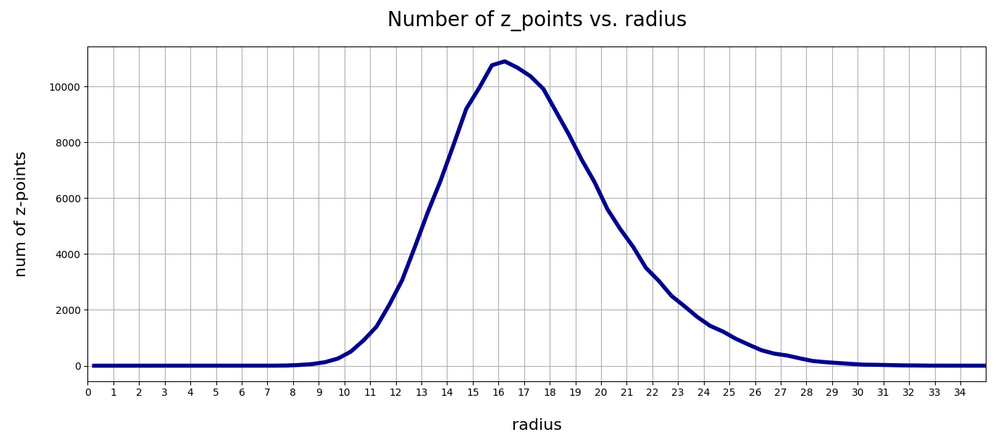So, there is some chance that the degrees of freedom associated with the logvar-layer and the statistical variation for epsilon may drive a VAE into other local minima or weight parameter ranges which do not lead to a z-point distribution around R = 16. But after the limitation of epsilon fluctuations all training runs found a loss minimum similar to the one of our simple AE – in the sense that it creates a z-point density distribution around R ≈ 16.

# VAE with tiny KL-loss: Inertia and clustering of the CelebA data?

Our VAE gives the following variation of the inertia vs. the number of assumed clusters: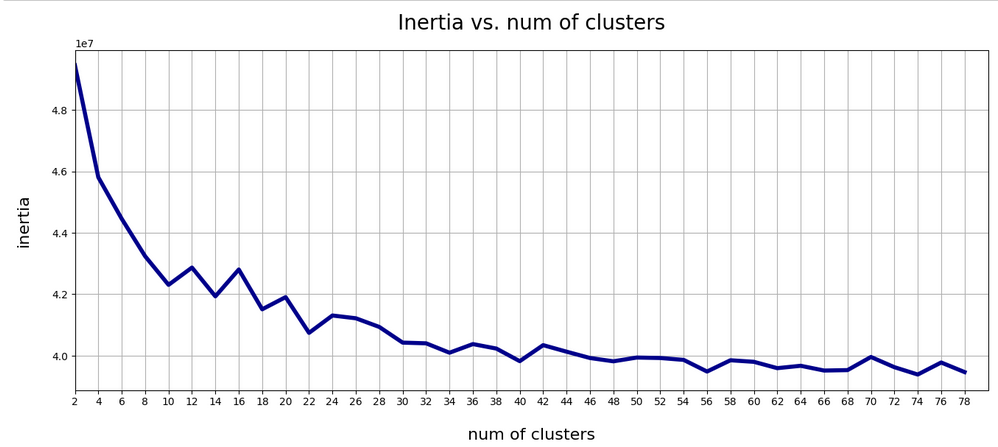This also looks pretty similar to one of the plots shown for our AE above.

# t-SNE for our VAE with a tiny KL loss

Below you find t-SNE plots for 20,000, 80,000 and 140,000 images:

Number of statistical z-points: 20,000 (non-standard t-SNE-parameters)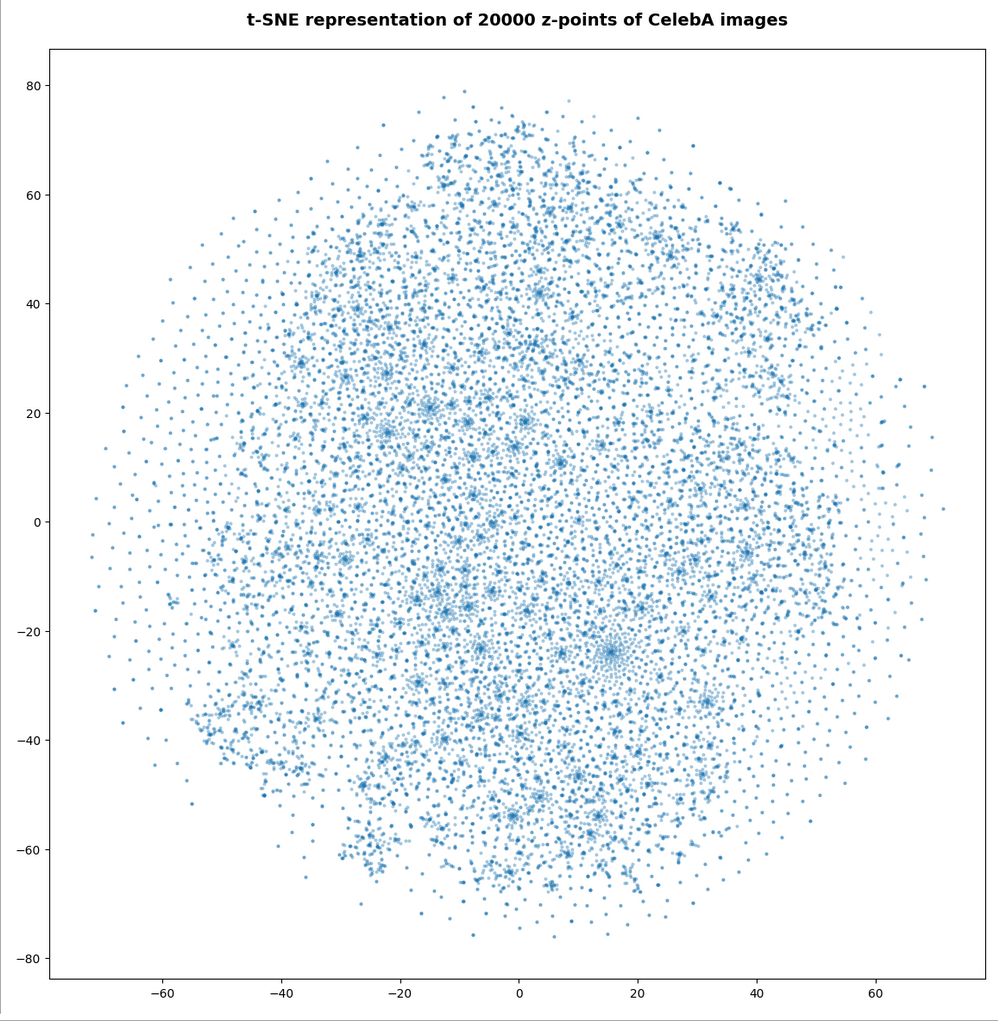This is quite similar to the related image for the AE. You just have to rotate it.

Number of statistical z-points: 80,000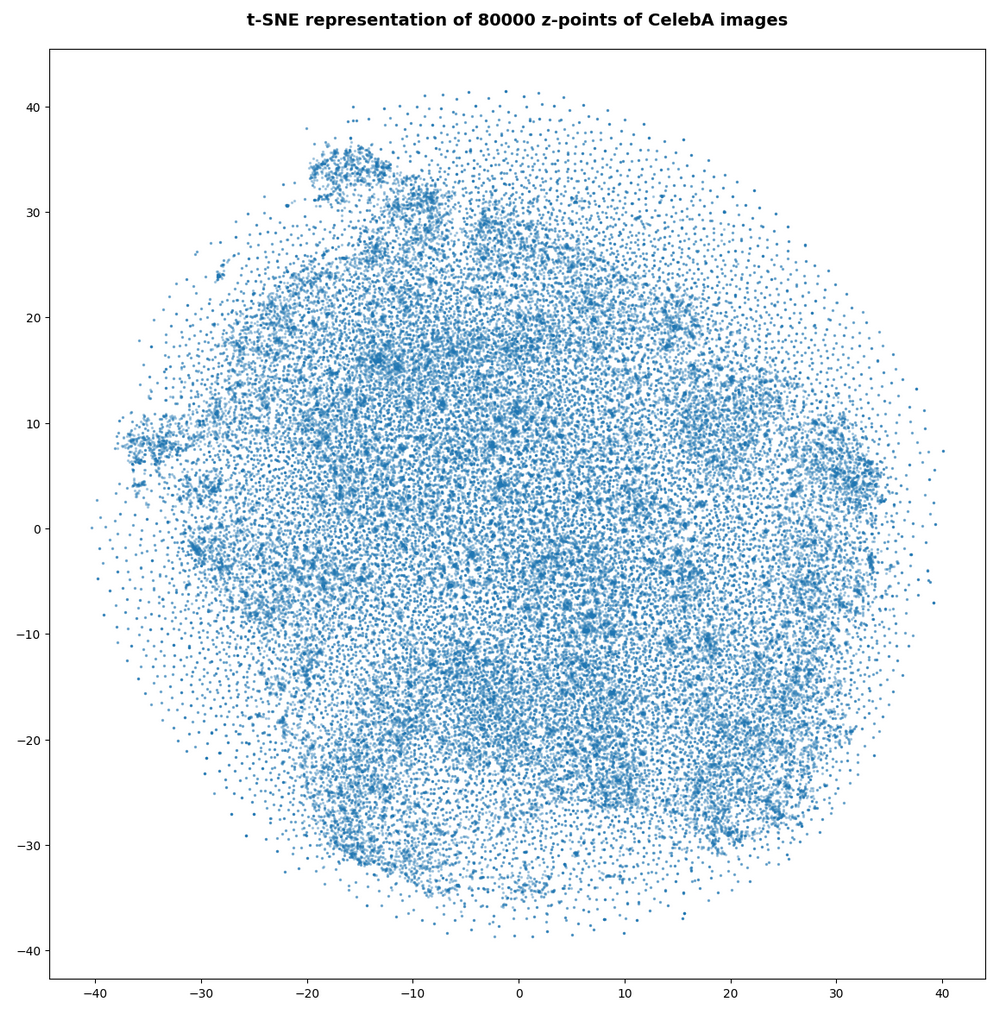Number of statistical z-points: 140,000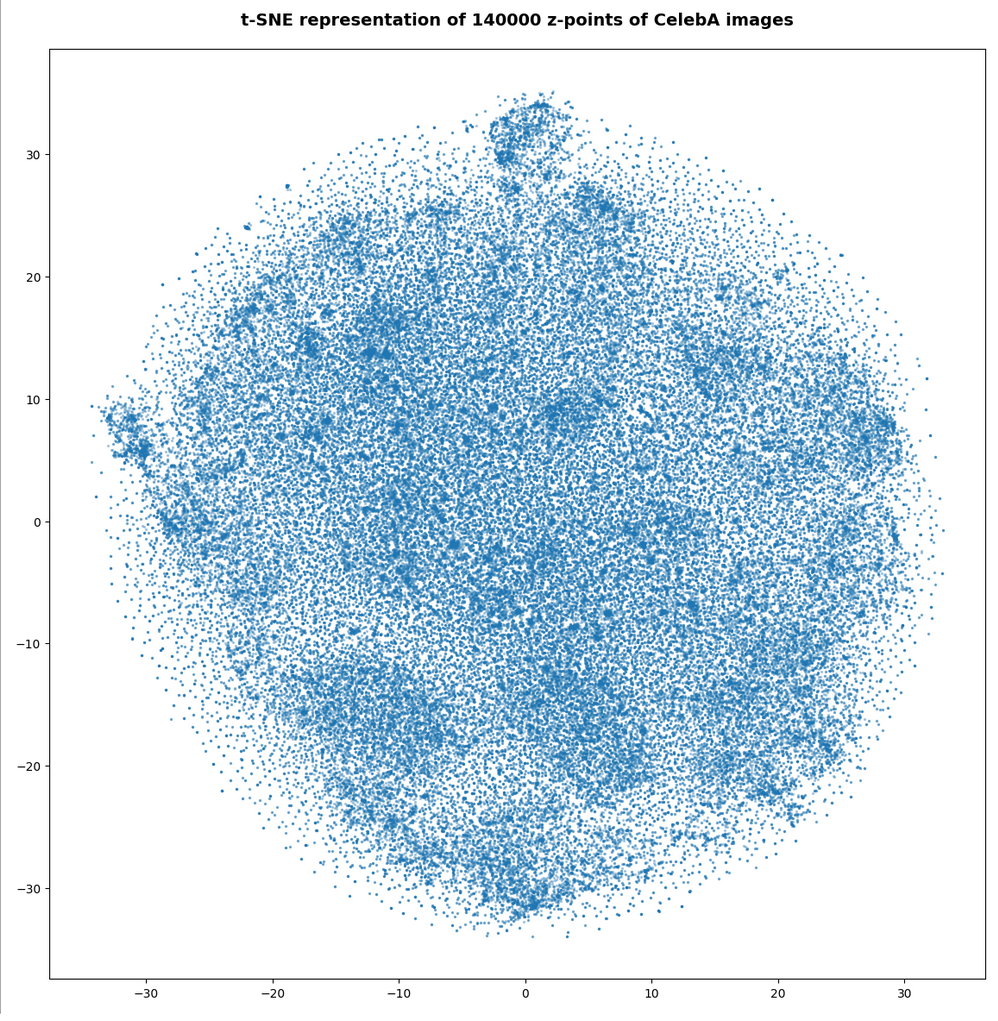All in all we get very similar indications as from our AE that some clustering is going on.

# VAE with tiny KL-loss: Should its logvar values become tiny, too?

Besides reproducing a similar z-point distribution with respect to radius values, is there another indication that a VAE behaves similar to an AE? What would be a clear sign that the similarity really exists on a deeper level of the layers and their weights?

The z-vector is calculated from the mu and logvar-vectors by:

```z = mu + exp(logvar/2)*epsilon
```

with epsilon coming from a normal distribution. Please note that we are talking about vectors of size z_dim=256 per image.

If a VAE with a tiny KL-loss really becomes similar to an AE it should define and set its z-points basically by using mu-values, only, and not by logvar-values. I.e. the VAE should become intelligent enough to ignore the degrees of freedom associated with the logvar-layer. Meaning that the z-point coordinates of a VAE with a very small Kl-loss should in the end be almost identical to the mu-component-values.

Ok, but to me it was not self-evident that a VAE during its training would learn

• to produce significant mu-related weight-values, only,
• and to keep the weight values for the connections to the logvar-layer so small that the logvar-impact on the z-space position gets negligible.

Before we speculate about reasons: Is there any evidence for a negligible logvar-contribution to the z-point coordinates or, equivalently, to the respective vector components?

# A VAE with tiny KL-loss produces tiny logvar values …

To get some quantitative data on the logvar impact the following steps are appropriate:

1. Get the size and algebraic sign of the logvar-values. Negative values logvar < -3 would be optimal.
2. Measure the deviation between the mu- and z_points vector components. There should only be a few components which show significant values &br; abs(mu – z) > 0.05
3. Compare the the radius-value determined by z-components vs. the radius values derived from mu-components, only, and measure the absolute and relative deviations. The relative deviation should be very small on average.

## Some values of logvar, (z – mu), z-radii and z-radius-deviations for a VAE with small KL-loss

Regarding the maximum value of the logvar’s vector-components I found

```3.4 ≥ max(logvar) ≥ -3.2. # for 1 up to 3 components out of a total 45.52 million components
```

The first value may appear to be big for a component. But typically there are only 2 (!) out of 170,000 x 256 = 43.52 million vector components in an interval of [-3, 5]. On the component level I found the following minimum, maximum and average-values

```Maximum value for logvar:  -2.0205276
Minimum value for logvar:  -24.660698
Average value for logvar:  -13.682616
```

The average value of logvar is pretty pleasing: Such big negative values indeed render the logvar-impact on the position of our z-points negligible. So we should only find very small deviations of the mu-components from the z-point components. And, actually, the maximum of the deviation between a z_point component and a mu component was delta_mu_z = 0.26:

```Maximum (z_points - mu) = delta_mu_z = 0.26  # on the component level
```

There were only 5 out of the 45.52 million components which showed an absolute deviation in the interval

```0.05 < abs(delta_mu_z) < 2.
```

The rest was much, much smaller!

What about radius values? Here the situation looks, of course, even better:

```max radius defined by z  :  33.10274
min radius defined by z  :  6.4961233
max radius defined by mu :  33.0972
min radius defined by mu :  6.494558

avg_z:      16.283989
avg_mu:     16.283972

max absolute difference :  0.018045425
avg absolute difference :  0.00094899256

max relative difference  :   0.00072147313
avg relative difference  :   6.1240215e-05
```

As expected, the relative deviations between z- and mu-based radius values became very small.

In another run (the one corresponding to the second density distribution curve above) I got the following values:

```Maximum value for logvar:  3.3761873
Minimum value for logvar:  -22.777826
Average value for logvar:  -13.4265175

avg_z:  17.37412
avg_mu: 17.374104

max delta rad relative :   0.012512478
avg delta rad relative :   6.5660715e-05
```

This tells us that the z-point distributions may vary a bit in their width, their precise center and average values. But, overall they appear to be similar. Especially with respect to a relative negligible contribution of logvar-terms to the z-point position. The relative impact of logvar on the radius value of a z-point is of the order 6.e-5, only.

All the above data confirm that a trained VAE with a very small KL-loss primarily uses mu-values to set the position of its z-points. During training the VAE moves along a path to an overall minimum on the loss hyperplane which leads to an area with weights that produce negligible logvar values.

# Explanation of the overall similarity of a VAE with tiny KL-loss to an AE

o far we can summarize: Under normal conditions the VAE’s behavior is pretty close to that of a similar AE. The VAE produces only small logvar values. z-point coordinates are extremely close to just the mu-coordinates.

Can we find a plausible reason for this result? Looking at the cost-hyperplane with respect to the Encoder weights helps:

The cost surface of a VAE spans across a space of many more weight parameters than a corresponding AE. The reason is that we have weights for the connection to the logvar-layer in addition to the weights for the mu-layer (or a single output layer as in a corresponding AE). But if we look at the corner of the weight-vector-space where the logvar-related values are pretty small, then we would at least find a local (if not global) loss minimum there for the same values of the mu-related weight parameters as in the corresponding AE (with mu replacing the z-output).

So our question reduces to the closely related question whether the old minimum of an AE remains at least a local one when we shift to a VAE – and this is indeed the case for the basic reason that the KL-contributions to the height of the cost-hyperplane are negligibly small everywhere (!) – even for higher logvar-related values.

This tells us that a gradient descent algorithm should indeed be able to find a cost minimum for very small values of logvar-related weights and for weight-values related to the mu-layer very close to the AE’s weight-values for direct connections to its output layer. And, of course, with all other weight parameter of the VAE-Decoder being close to the values of the weights of a corresponding AE. At least under the condition that all variable quantities really change smoothly during training.

# Does a VAE with small KL-loss produce reasonable face images?

A last test to confirm that a VAE with a very small KL-loss operates as an comparable AE is a trial to create images with recognizable human faces from randomly chosen points in z-space. Such a trial should fail! I just show you three results – one for a normal distribution of the z-point components. And two for equidistant distribution of component values up to 3, 8 and 16:

z-point coordinates from normal distribution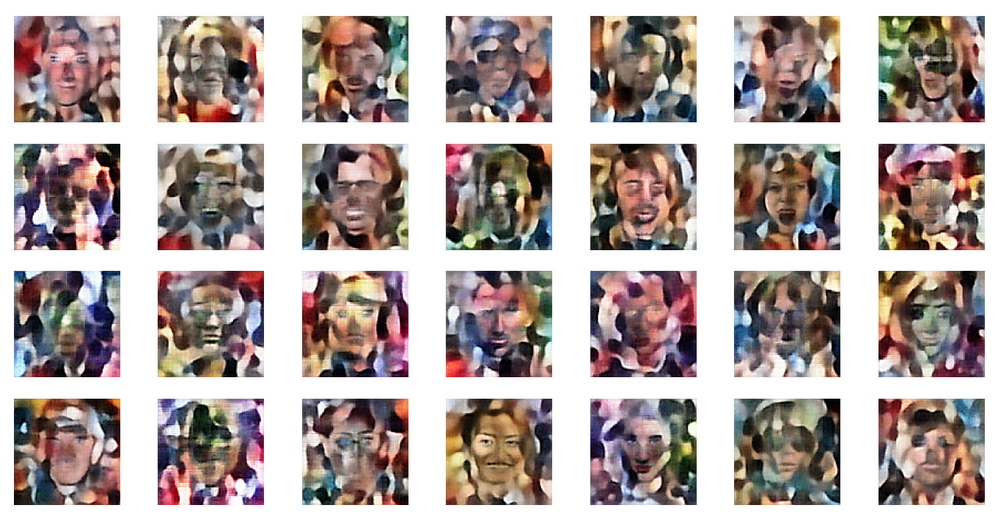z-point coordinates from equidistant distribution in [-2,2]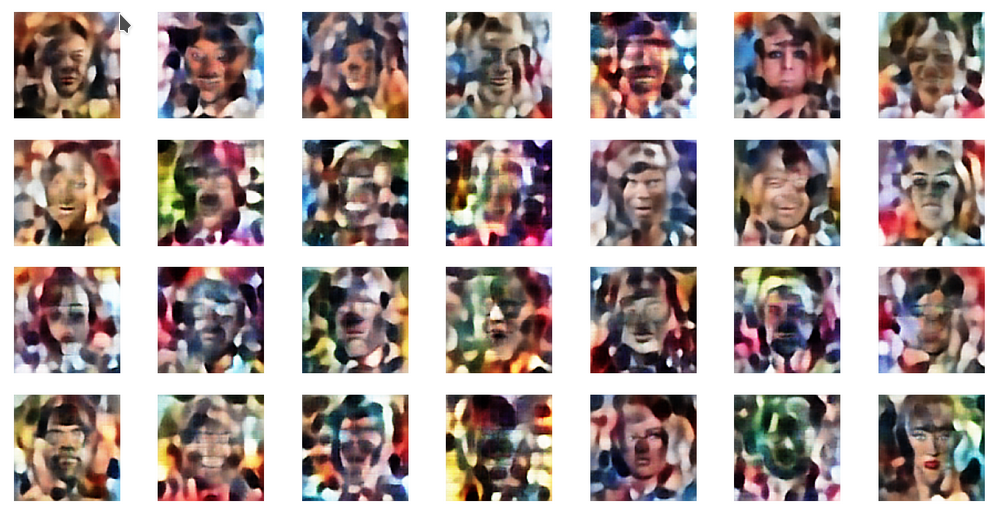z-point coordinates from equidistant distribution in [-10,10]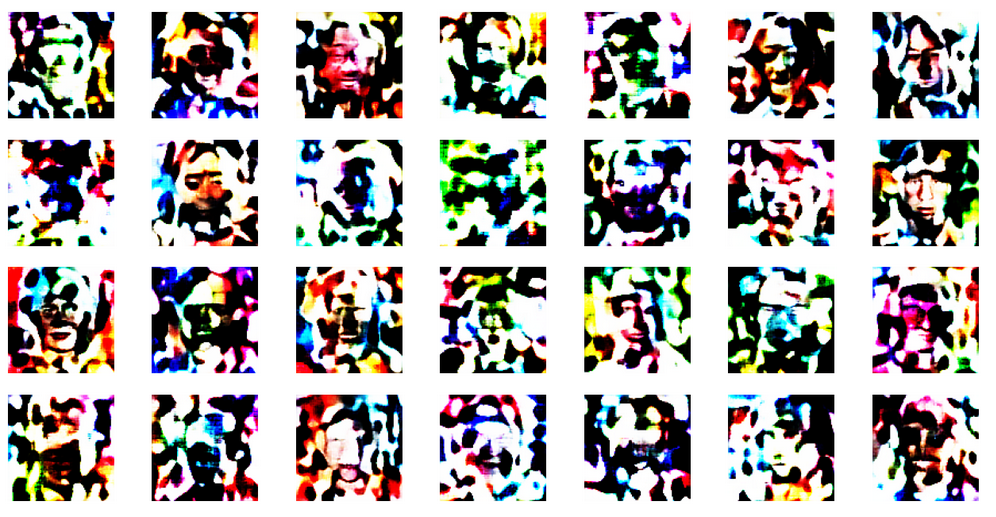This reminds us very much about the behavior of an AE. See: Autoencoders, latent space and the curse of high dimensionality – I.

The z-point distribution in latent space of a VAE with a very small KL-loss obviously is as complicated as that of an AE. Neighboring points of a z-point which leads to a good image produce chaotic images. The transition path from good z-points to other meaningful z-points is confined to a very small filament-like volume.

# Conclusion

A trained VAE with only a tiny KL-loss contribution will under normal circumstances behave similar to an AE with a the same hidden (convolutional) layers. It may, however, be necessary to limit the statistical variation of the epsilon factor in the z-point calculation based on mu– and logvar-values.

The similarity is based on very small logvar-values after training. The VAE creates a z-point distribution which shows the same dependency on the radius as an AE. We see similar indications and patterns of clustering. And the VAE fails to produce human faces from random z-points in the latent space – as a comparable AE.

We have found a plausible reason for this similarity by comparing the minimum of the loss hyperplane in the weight-loss parameter space with a corresponding minimum in the weight-loss space of the VAE – at a position with small weights for the connection to the logvar layers.

The z-point density distribution shows a maximum at a radius between 16 and 17. The z-point distribution basically has a Gaussian form. In the next post we shall look a bit closer at these findings – and their origin in Gaussian distributions along the coordinate axes of the latent space. After an application of a PCA analysis we shall furthermore see that the z-point distribution in an AE’s latent vector space is indeed fragmented and shows filaments on certain length scales. A VAE with a tiny KL-loss will show the same fragmentation.

In further forthcoming posts we shall afterward investigate the confining and at the same time blurring impact of the KL-loss on the latent space. Which will make it usable for creative purposes. But the next post

will first show you how to change model parameters at inference time.

And let us all who praise freedom not forget:
The worst fascist, war criminal and killer living today is the Putler. He must be isolated at all levels, be denazified and sooner than later be imprisoned. Long live a free and democratic Ukraine!